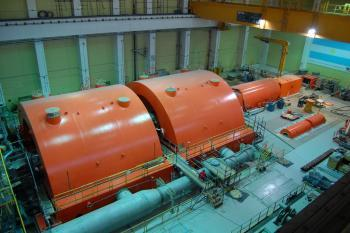# Nuclear Energy# Electric generatorThe electric generator is an essential element in nuclear power plants. The objective of nucelar power plants is to convert nuclear energy from nuclear fission reactions into electrical energy. The function of the electric generator is to convert the mechanical energy from one coming from a steam turbine into electricity.

### What is an electric generator?

An electric generator is a machine capable of transforming some type of energy, which can be chemical energy, mechanical or light energy, into electrical energy. Mechanical energy sources include steam turbines, gas turbines, water turbines, internal combustion engines and even cranks.

An electric generator is any device capable of maintaining an electrical potential difference (voltage) between two points, called poles or terminals. Electric generators are electrical machines designed to transform mechanical energy into electrical energy.

In the generation of electricity, a generator is a device that converts motive power (mechanical energy) into electrical energy for use in an external circuit. The first electromagnetic generator, the Faraday disc, was invented in 1831 by the British scientist Michael Faraday. The generators provide almost all the power for the electrical networks.

## Operation of an electric generator

The operation of an electric generator is similar to that of an electric motor running in reverse.

This transformation is achieved by the action of a magnetic field on the electrical conductors arranged in coils on a sheet metal frame. If mechanically there is a relative movement between the conductors and the field, an electromotive force is generated, as physicist Michael Faraday discovered.

The inverse conversion of electrical energy into mechanical energy is done by an electric motor. For this reason, electric motors and electric generators have many similarities. Many electric motors can be mechanically driven to generate electricity and often make manual generators acceptable.

## Modeling an electric generator

A real generator can be modeled in two different ways:

• An ideal voltage generator with series resistance.
• An ideal current generator with a resistor connected in parallel.

### Ideal voltage generator

The ideal voltage generator is a theoretical model. It is a dipole capable of imposing a constant voltage regardless of the load connected to its terminals. It is also called a voltage source.

In an open circuit, the voltage that exists at its terminals when no current is supplied is the no-load voltage. Therefore, the voltage generator is a virtual dipole whose voltage at its terminals is always equal to the no-load voltage, regardless of the value of the current output.

The voltage generator can only be a theoretical model because, due to a short circuit, it must deliver an infinite current and, therefore, provide an infinite power that is unattainable.

### Ideal current generator

For the ideal current generator, the current produced is constant, regardless of the voltage required and the load that must be supplied.

It is also a theoretical model because the opening of a circuit comprising a non-zero current generator should provide infinite power.

The actual generators can be modeled simply by combining an ideal current generator and a resistor connected in parallel. Such a model is called the Norton model.

An inductive dipole is a transient current generator that opposes any variation in the intensity of the current flowing through it. When a circuit comprising an inductive dipole crossed by a non-zero current is opened, a high voltage can appear that can generate an electric arc on the switch.

## Ideal electric generation

In the theoretical study of electrical phenomena and circuits, generators are generally considered ideal. An ideal generator can produce any voltage and current without any limit and is free of internal resistance. The value of the current or voltage generated is independent of the applied load.

In reality, there are no ideal generators, since any device has its intrinsic internal resistance, and is capable of generating voltage and current only within certain limits.

A real voltage generator can be represented as an ideal voltage generator with serialized internal resistance, while the actual current generator can be represented as an ideal current generator in parallel with the internal resistance.

In theory, if a circuit comprising an ideal current generator is opened, the output voltage should increase to infinity. However, in real generators there is a voltage limit value above which the current collapses to zero. From the theoretical point of view, in the theoretical representation of the real generator, the current output of the ideal generator is closed in the internal resistance.

## Electric generators and nuclear energy

Electric generators are of vital importance in nuclear power plants.

Once the nuclear power plant has started to turn the turbine, the electric generator is in charge of converting the mechanical energy of the turbine into electrical energy.